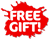ISBN: 978-1-988586-72-4

## Level 2 Systems of Equations 2.14 Learning WorkbookVaughan Mitchell and Tom Sidebotham

• 895

Level 2 Systems of Equations Learning Workbook covers NCEA Level 2 Achievement Standard 91269 (Mathematics and Statistics 2.14): Apply systems of equations in solving problems.
This standard is internally assessed and worth 2 credits.
The workbook features:
• bite-sized sections of notes with student-friendly explanations
• worked examples to illustrate fundamental concepts
• plenty of exercises so students can improve their skills and practise problem solving
• answers to all exercises, which are provided at the end of the book
• practice assessment tasks with fully worked solutions.
An index gives easy access to important terms and definitions highlighted in the text.
This workbook covers the forming and using of pairs of simultaneous equations, and systems of linear inequations, in order to solve problems. Different representations of equations and inequations are discussed and solutions to practical problems are interpreted in context.
The concise notes and write-on exercises keep student work together in a compact format, providing a useful end-of-topic revision tool.
This workbook is ideal for use in the classroom or for homework, home study and revision.

Published: 10/2020